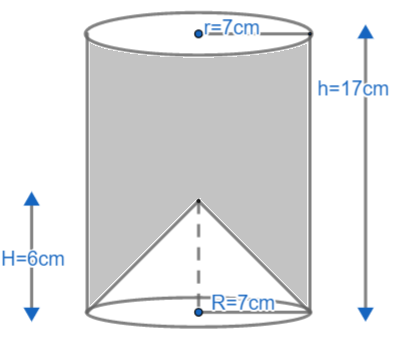A cylindrical glass of radius 7cm is filled with mango juice up to a height of 17cm. When carefully observed, the bottom of glass is observed by a transparent cone of height 6cm and radius 7cm. ____ is the actual value of juice.

# A cylindrical glass of radius 7cm is filled with mango juice up to a height of 17cm. When carefully observed, the bottom of glass is observed by a transparent cone of height 6cm and radius 7cm. ____ is the actual value of juice.

Fill Out the Form for Expert Academic Guidance!l

+91

Live ClassesBooksTest SeriesSelf Learning

Verify OTP Code (required)

### Solution:

The radius and height of the cone and cylinder are given here. A cone is placed between the cylinder and the glass is formed. The radius of the cylinder is 7 cm and the height of the cylinder is 17 cm. So let r = 7 cm and h = 17 cm.
The radius of the cone is 7 cm and the height of the cone is 6 cm. So let R = 7 cm and H = 6 cm.
Let's draw a diagram to clearly understand the shape of the glass.Now we need to find the actual volume of the juice, which means we need to find the volume of the shaded part of the glass. As we can see from the diagram, the volume of the shaded part of the glass will be the difference between the volume of the cylindrical part and the volume of the conical part. So let's find the volume of a cylinder that has r = 7 cm, h = 17 cm and the volume of a cone that has R = 7 cm and H = 6 cm.
The volume of the cylinder is given byThe volume of the cylindrical part of the glass will be

Volume of cone is given by  . So,
Volume of cone part of glass will be,

Now we know that, Volume of glass = volume of cylindrical part - volume of conical part.
So, Volume of glass
.
Hence, actual volume of juice will be   .

## Related content

 Area of Square Area of Isosceles Triangle Pythagoras Theorem Triangle Formula Perimeter of Triangle Formula Area Formulae Volume of Cone Formula Matrices and Determinants_mathematics Critical Points Solved Examples Type of relations_mathematics+91

Live ClassesBooksTest SeriesSelf Learning

Verify OTP Code (required)